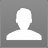Categoty :
elaine : Solve the inequality x2 + 2x > 15 and choose the graph for the solution set.: Solve the inequality x2 + 2x > 15 and choose the graph for the solution set. {x: x < - 5 or x > 3}, Graph 4#0#{x: - 5 ≤ x ≤ 3}, Graph 3#0#{x: x = - 5 and x = 3}, Graph 2#0#{x: x ≤ - 5 or x ≥ 3}, Graph 1#0#